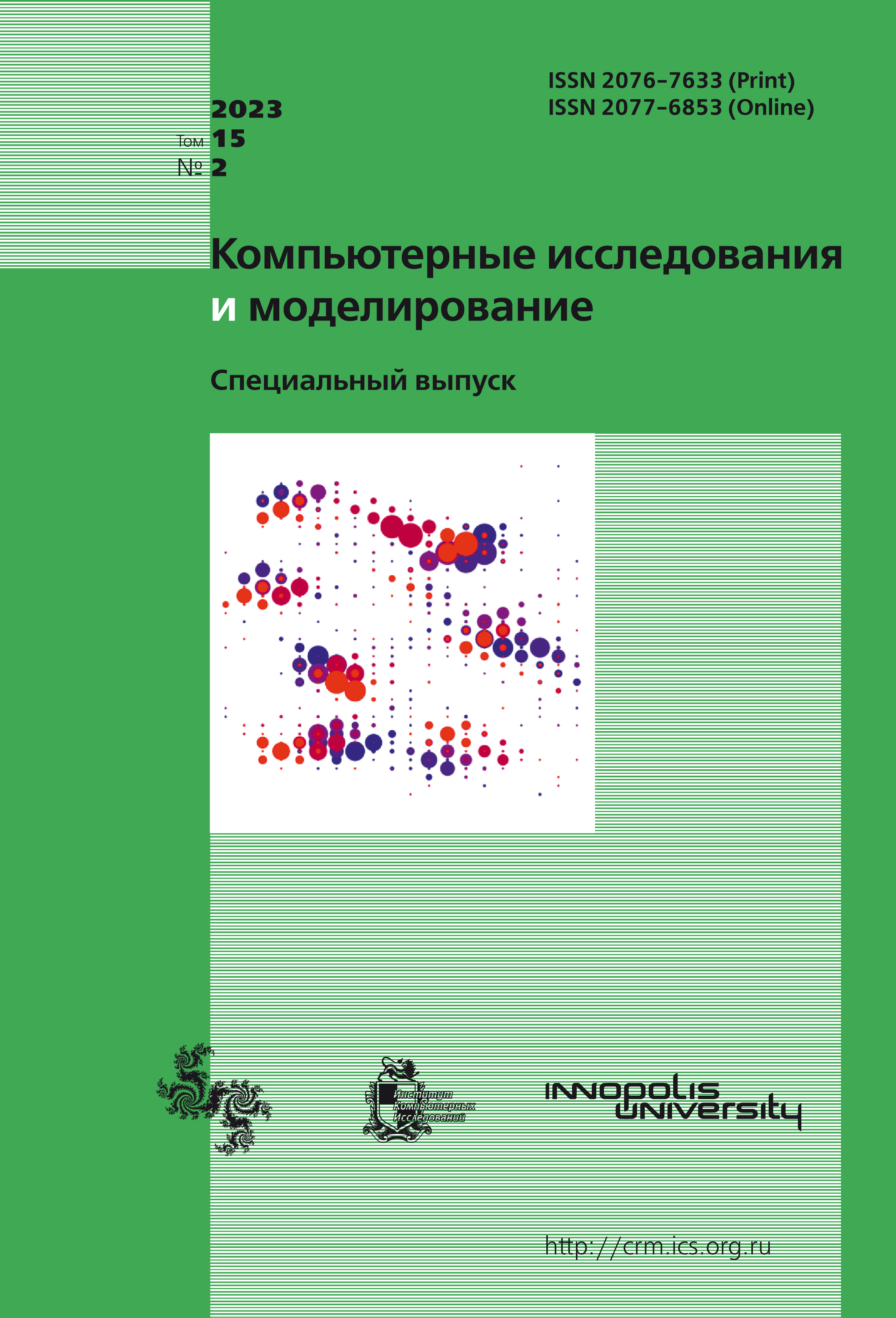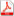Latest issue Issue 2, 2023 Vol. 15

# All issues

Transport modeling: averaging price matricespdf (762K)

This paper considers various approaches to averaging the generalized travel costs calculated for different modes of travel in the transportation network. The mode of transportation is understood to mean both the mode of transport, for example, a car or public transport, and movement without the use of transport, for example, on foot. The task of calculating the trip matrices includes the task of calculating the total matrices, in other words, estimating the total demand for movements by all modes, as well as the task of splitting the matrices according to the mode, also called modal splitting. To calculate trip matrices, gravitational, entropy and other models are used, in which the probability of movement between zones is estimated based on a certain measure of the distance of these zones from each other. Usually, the generalized cost of moving along the optimal path between zones is used as a distance measure. However, the generalized cost of movement differs for different modes of movement. When calculating the total trip matrices, it becomes necessary to average the generalized costs by modes of movement. The averaging procedure is subject to the natural requirement of monotonicity in all arguments. This requirement is not met by some commonly used averaging methods, for example, averaging with weights. The problem of modal splitting is solved by applying the methods of discrete choice theory. In particular, within the framework of the theory of discrete choice, correct methods have been developed for averaging the utility of alternatives that are monotonic in all arguments. The authors propose some adaptation of the methods of the theory of discrete choice for application to the calculation of the average cost of movements in the gravitational and entropy models. The transfer of averaging formulas from the context of the modal splitting model to the trip matrix calculation model requires the introduction of new parameters and the derivation of conditions for the possible value of these parameters, which was done in this article. The issues of recalibration of the gravitational function, which is necessary when switching to a new averaging method, if the existing function is calibrated taking into account the use of the weighted average cost, were also considered. The proposed methods were implemented on the example of a small fragment of the transport network. The results of calculations are presented, demonstrating the advantage of the proposed methods.

Keywords: multinomial logit, discrete choice model, modal choice, gravity function
Citation in English: Podlipnova I.V., Persiianov M.I., Shvetsov V.I., Gasnikova E.V. Transport modeling: averaging price matrices // Computer Research and Modeling, 2023, vol. 15, no. 2, pp. 317-327
Citation in English: Podlipnova I.V., Persiianov M.I., Shvetsov V.I., Gasnikova E.V. Transport modeling: averaging price matrices // Computer Research and Modeling, 2023, vol. 15, no. 2, pp. 317-327
DOI: 10.20537/2076-7633-2023-15-2-317-327

Full-text version of the journal is also available on the web site of the scientific electronic library eLIBRARY.RU

The journal is included in the Russian Science Citation Index

The journal is included in the List of Russian peer-reviewed journals publishing the main research results of PhD and doctoral dissertations.

International Interdisciplinary Conference "Mathematics. Computing. Education"

The journal is included in the RSCI

Indexed in Scopus